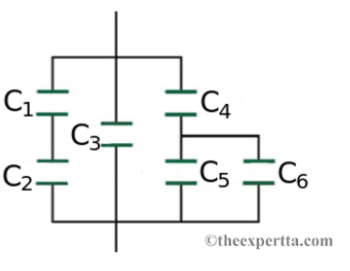# Problem: A combination of series and parallel connections of capacitors is shown in the figure. The sizes of these capacitors are given by the following data:C1 = 5.4 μFC2 = 3.7 μFC3 = 8.1 μFC4 = 1.2 μFC5 = 0.65 μFC6 = 14 μFFind the total capacitance of the combination of capacitors in microfarads

###### FREE Expert Solution

Equivalent capacitance for series connection:

$\overline{)\frac{\mathbf{1}}{{\mathbf{C}}_{\mathbf{e}\mathbf{q}}}{\mathbf{=}}\frac{\mathbf{1}}{{\mathbf{C}}_{\mathbf{1}}}{\mathbf{+}}\frac{\mathbf{1}}{{\mathbf{C}}_{\mathbf{2}}}{\mathbf{+}}{\mathbf{.}}{\mathbf{.}}{\mathbf{.}}{\mathbf{+}}\frac{\mathbf{1}}{{\mathbf{C}}_{\mathbf{n}}}}$

Equivalent capacitance for parallel connection:

$\overline{){{\mathbf{C}}}_{\mathbf{e}\mathbf{q}}{\mathbf{=}}{{\mathbf{C}}}_{{\mathbf{1}}}{\mathbf{+}}{{\mathbf{C}}}_{{\mathbf{2}}}{\mathbf{+}}{\mathbf{.}}{\mathbf{.}}{\mathbf{.}}{\mathbf{+}}{{\mathbf{C}}}_{{\mathbf{n}}}}$

C1 and C2 are in series.

1/C12 = 1/5.4 + 1/3.7 = 0.455455455

C12 = 2.2  μF

C5 and C6 are in parallel.

C56 = 0.65 + 16 = 16.65 μF

80% (339 ratings)###### Problem Details

A combination of series and parallel connections of capacitors is shown in the figure. The sizes of these capacitors are given by the following data:

C1 = 5.4 μF

C2 = 3.7 μF

C3 = 8.1 μF

C4 = 1.2 μF

C5 = 0.65 μF

C6 = 14 μFFind the total capacitance of the combination of capacitors in microfarads

Frequently Asked Questions

What scientific concept do you need to know in order to solve this problem?

Our tutors have indicated that to solve this problem you will need to apply the Capacitors & Capacitance concept. You can view video lessons to learn Capacitors & Capacitance. Or if you need more Capacitors & Capacitance practice, you can also practice Capacitors & Capacitance practice problems.

What professor is this problem relevant for?

Based on our data, we think this problem is relevant for Professor Jerousek's class at UCF.